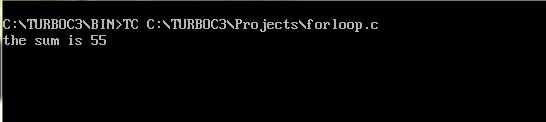UncleCoder.com

UncleCoder.com

Free programming examples and instructions

# C program to find sum of first 10 integers using for loop

## C program for how to find sum of first 10 integers using for loop

by Krishna

Posted on 27 Jul 2018 Category: C Views: 3171

C program to find sum of first 10 integers using for loop

A loop is used for executing a block of statements repeatedly until a given condition returns false.
In this section we will write a sample for loop program to find the sum of first 10 natural numbers.
The syntax for 'for loop' is shown below

``````for ( initialization; condition; increment ) {
statement(s);
}``````

The initialization statement is executed only once.
Then, the test expression is evaluated. If the test expression is evaluated to false, the for loop is terminated.
However, if the test expression is evaluated to true, statements inside the body of for loop are executed, and the update expression is updated.
Again the test expression is evaluated.
This process goes on until the test expression is false. When the test expression is false, the loop terminates.

``````#include <stdio.h>
#include<conio.h>
void main()
{
int  j, sum = 0;

for (j = 1; j <= 10; j++)
{
sum = sum + j;
printf("%d ",j);
}
printf("\nthe sum is %d\n", sum);
getch();
}``````

OUTPUTRelated Articles

C Program to add two numbers

C program to find factorial of a number using function

C program to generate Star sequence

C Program to find factorial of a Number using While Loop

C Program to Generate Multiplication Table

C program to find largest among two numbers

C program to print Fibonacci series up to 100

C Program to find sum of two numbers using Function

C Program to find sum of digits

C program to find the factors of a number